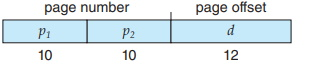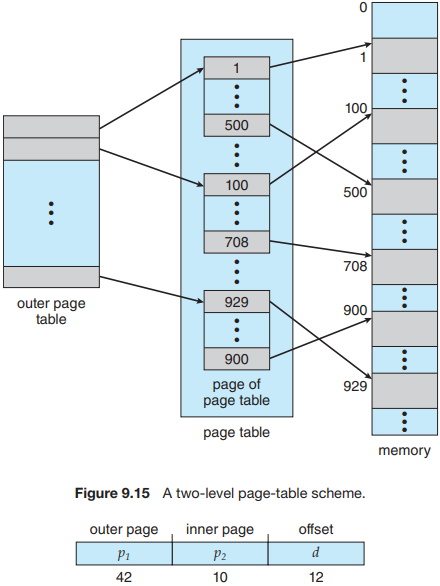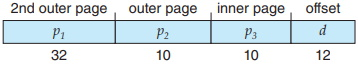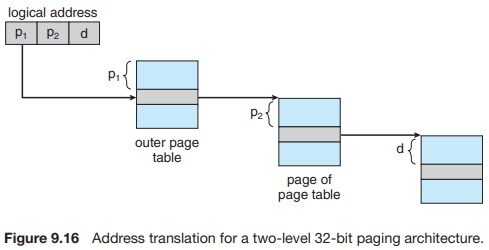#Operating System Memory Paging - Page Table StructureMost modern computer systems support a large logical address space (232 to 264). In such an environment, the page table itself becomes excessively large. For example, consider a system with a 32-bit logical address space. If the page size in such a system is 4 KB (212), then a page table may consist of over 1 million entries (220 = 232/212). Assuming that each entry consists of 4 bytes, each process may need up to 4 MB of physical address space for the page table alone. Clearly, we would not want to allocate the page table contiguously in main memory. One simple solution to this problem is to divide the page table into smaller pieces. We can accomplish this division in several ways.

One way is to use a two-level paging algorithm, in which the page table itself is also paged (Figure 9.15). For example, consider again the system with a 32-bit logical address space and a page size of 4 KB. A logical address is divided into a page number consisting of 20 bits and a page offset consisting of 12 bits. Because we page the page table, the page number is further divided into a 10-bit page number and a 10-bit page offset. Thus, a logical address is as follows:where p1 is an index into the outer page table and p2 is the displacement within the page of the inner page table. The address-translation method for this architecture is shown in Figure 9.16. Because address translation works from the outer page table inward, this scheme is also known as a forward-mapped page table.

For a system with a 64-bit logical address space, a two-level paging scheme is no longer appropriate. To illustrate this point, let’s suppose that the page size in such a system is 4 KB (212). In this case, the page table consists of up to 252 entries. If we use a two-level paging scheme, then the inner page tables can conveniently be one page long, or contain 210 4-byte entries. The addresses look like this:The outer page table consists of 242 entries, or 244 bytes. The obvious way to avoid such a large table is to divide the outer page table into smaller pieces. (This approach is also used on some 32-bit processors for added flexibility and efficiency.)

We can divide the outer page table in various ways. For example, we can page the outer page table, giving us a three-level paging scheme. Suppose that the outer page table is made up of standard-size pages (210 entries, or 212 bytes). In this case, a 64-bit address space is still daunting:The outer page table is still 234 bytes (16 GB) in size.

The next step would be a four-level paging scheme, where the second-level outer page table itself is also paged, and so forth. The 64-bit UltraSPARC would require seven levels of paging - a prohibitive number of memory accesses -to translate each logical address. You can see from this example why, for 64-bit architectures, hierarchical page tables are generally considered inappropriate.